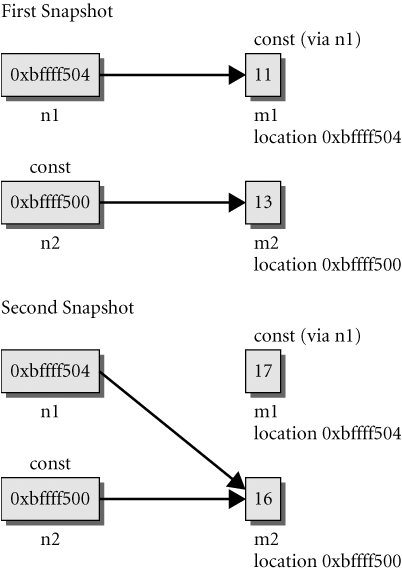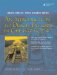# const* and *const

Suppose that we have a pointer ptr that is storing the address of a variable

```vbl:Type* ptr = &vbl;
```

When using a pointer, two objects are involved: the pointer itself and the object pointed to. This means there are two possible layers of protection that we might want to impose with const:

1. If we want to make sure that ptr cannot point to any other memory location, we can write it one of two ways:

```Type* const ptr = &vbl;
Type* const ptr(&vbl);
```

The pointer is a const but the addressed object can be changed.

2. If we want to make sure that the value of vbl cannot be changed by dereferencing ptr, we can write it in two ways:

```const Type* ptr = &vbl;
const Type* ptr(&vbl);
```

In this case, the addressed object is a constant but the pointer is not.

In addition, if we want to impose both kinds of protection we can write:

```const Type* const ptr = &vbl;
const Type* const ptr(&vbl);
```

Here is a good way to remember which is which: Read each of the following definitions from right to left (starting with the defined variable).

```const char * x = &p; /* x is a pointer to const char*/
char * const y = &q; /* y is a const pointer to char */
const char * const z = &r; /* z is a const pointer to a const char */
```

A short program that demonstrates the two kinds of protection is shown in Example 1.22.

Example 1.22. src/constptr/constptr.cpp

 ```#include using namespace std; int main() { int m1(11), m2(13); const int* n1(&m1); int* const n2(&m2); // First snapshot cout << "n1 = " << n1 << ' ' << *n1 << ' ' << "n2 = " << n2 << ' ' << *n2 << endl; n1 = &m2; //*n1 = 15; <-- 1 m1 = 17; <-- 2 //n2 = &m1; <-- 3 *n2 = 16; <-- 4 // Second snapshot cout << "n1 = " << n1 << ' ' << *n1 << ' ' << "n2 = " << n2 << ' ' << *n2 << endl; return 0; } Output: src/constptr> g++ constptr.cpp src/constptr> ./a.out n1 = 0xbffff504 11 n2 = 0xbffff500 13 n1 = 0xbffff500 16 n2 = 0xbffff500 16 src/constptr> ```   (1)error: assignment of read-only location (2)m2 is an ordinary int variableOK to assign (3)error: assignment of read-only variable 'n2' (4)okay to change target

Figure 1.2 shows two snapshots of memory that may help to clarify what is happening when the program runs. Notice that the program produces a memory leak.

Figure 1.2. Two snapshots of memory showing what the program in Example 1.2 producesAn object that is read-only when accessed through one pointer may be changeable when accessed through another pointer. This fact is commonly exploited in the design of functions.

```char* strcpy(char* dst, const char* src); // strcpy cannot change *src
```

It is okay to assign the address of a variable to a pointer to const. It is an error to assign the address of a const object to an unrestricted (i.e., non-const) pointer variable because that would allow the const object's value to be changed.

```int a = 1;
const int c = 2;
const int* p1 = &c; // OK
const int* p2 = &a; // OK
int* p3 = &c; // error
*p3 = 5; // error
```

It is good programming practice to use const to protect pointer and reference parameters that do not need to be altered by the action of a function. Read-only reference parameters provide the power and efficiency of pass-by-reference with the safety of pass-by-value (see Section 5.6).An Introduction to Design Patterns in C++ with Qt 4
ISBN: 0131879057
EAN: 2147483647
Year: 2004
Pages: 268